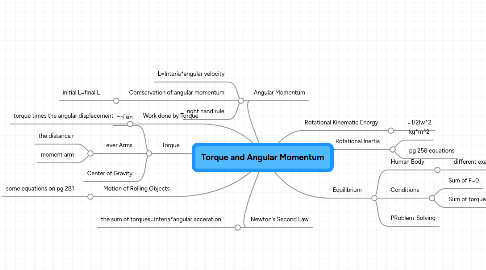# Torque and Angular Momentum

Get Started. It's FreeTorque and Angular Momentum## 1. Torque

### 1.2. Lever Arms

1.2.1. the distance r

1.2.2. moment arm

## 5. Angular Momentum

### 5.2. Comservation of angular momentum

5.2.1. initial L=final L

## 8. Equilibrium

### 8.1. Human Body

8.1.1. different examples

### 8.2. Conditions

8.2.1. Sum of F=0

8.2.2. Sum of torques=o